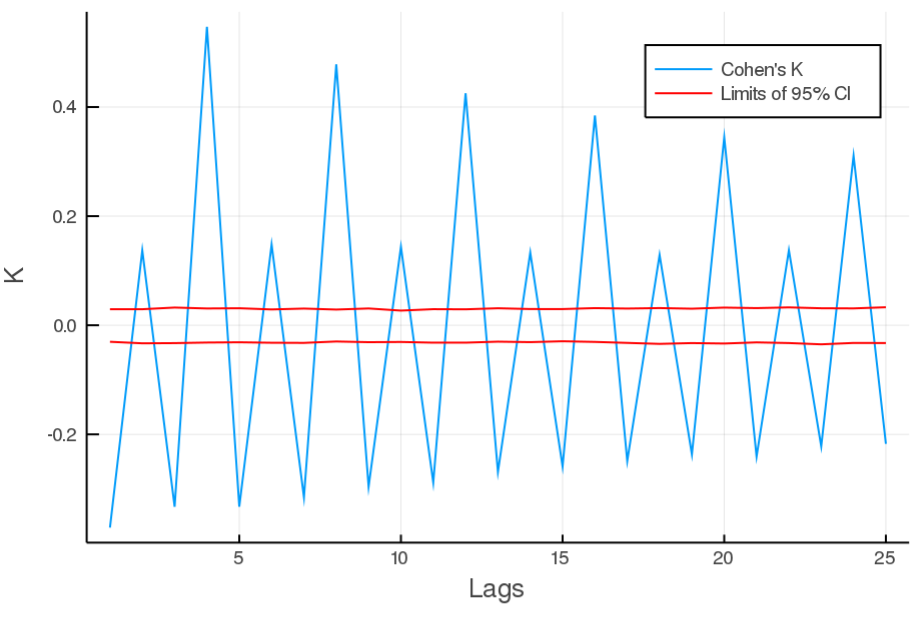## SerialDependence.jl

Julia module containing standard methods from categorical time-series analysis.
Author CNelias
Popularity
3 Stars
Updated Last
1 Year Ago
Started In
February 2019

# SerialDependence.jl

When dealing with categorical data, things like autocorrelation function are not defined. This is what this module is for : computing categorical serial dependences.

The module mostly implements the methods described in C. Weiss's book "An Introduction to Discrete-Valued Time Series" (2018) , with some extras. It contains three main functions :

## Main functions

All the module's functions require a `'lag's` input : `'lags'` can be an `Int`, or an `Array{Int,1}` if you want to do a serial dependence plot. The function then returns a `Float64` or an `Array{Float64,1}` depending on `'lags'` being an `Int` or `Array{Int,1}`.

• `cramer_coefficient(series, lags)`: measures average association between elements of `'series'` at time `t` and time `t + lags`. Cramer's k is an unsigned measurement : its values lies in [0,1], 0 being perfect independence and 1 perfect dependence. k can be bias, for more infos, refer to .

• `cohen_coefficient(series, lags)`: measures average agreement between elements of `'series'` at time `t` and time `t + lags`.
Cohen's k is a signed measurement : its values lie in [-pe/(1 -pe), 1], with positive (negative) values indicating positive (negative) serial dependence at 'lags'. pe is probability of agreement by chance.

• `theils_u(series, lags)`: measures average portion of information known about `'series'` at `t + lags` given that `'series'` is known at time `t`. U is an unsigned measurement: its values lies in [0,1], 0 meaning no information shared and 1 complete knowledge (determinism).

### Usefull extras

• `bootstrap_CI(Series, lags, coef_func, n_iter = 1000)`: Returns top and bottom limit for a 95% confidence interval at values of 'lags'.
• `'coef_func'` is the function for which the CI needs to be computed. Possible values : 'cramer_coefficient, cohen_coefficient, theils_u'.
• `'n_iter'` controls how many iterations are run during the bootstrap process. Large `'n_iter'`, means more precision but also more compute time.
• `rate_evolution(Series)`: This is a visual test of "stationarity" : if it varies linearly, then the time-series can be considered as stationary. Returns an `array` of `array`. Each of the internal array represents one of the categories in `'Series'`and describes it's evolution rate.

## Example

Using the pewee birdsong data (1943) one can do a serial dependence plot using Cohen's cofficient as follow :

```using DelimitedFiles
using SerialDependence
using Plots
lags = collect(1:25)
v = cohen_coefficient(series, lags)
t, b = bootstrap_CI(series, cramer_coefficient, lags)
a = plot(lags, v, xlabel = "Lags", ylabel = "K", label = "Cramer's k")
plot!(a, lags, t, color = "red", label = "Limits of 95% CI"); plot!(a, lags, b, color = "red", label = "")```## TO-DO

[] Implement bias correction for cramer's v

 DOI : 10.1002/9781119097013

### Used By Packages

No packages found.